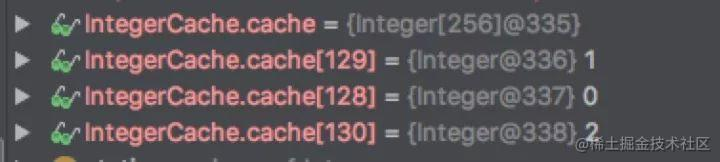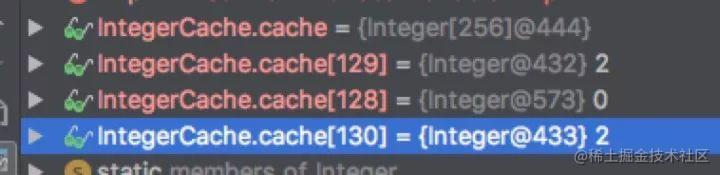你真的了解Java的形参和实参吗？1. public    class Main {

2.    public static void main(String[] args) {

3.        Integer a = 1;

4.        Integer b = 2;

5.        System.out.println("a=" + a + ",b=" + b);

6.        swap(a, b);

7.        System.out.println("a=" + a + ",b=" + b);

8.    }

9.    private static void swap(Integer numa, Integer numb) {

10.        //请实现

11.    }

12. }

1. public static void main( String[] args ) {

2.         Integer a = 1;

3.         Integer b = 2;

4.         System.out .println( "a=" + a + ",b=" + b);

5.         Integer tmp = a;

6.        a = b;

7.        b = tmp;

8.         System.out .println( "a=" + a + ",b=" + b);

9.     }

1. a=1,b=2

2. a=2,b=1

1. private static void swap(Integer numa, Integer numb) {

2.        Integer tmp = numa;

3.        numa = numb;

4.        numb = tmp;

5.        System.out.println("numa=" + numa + ",numb=" + numb);

6. }

1. a=1,b=2

2. numa=2,numb=1

3. a=1,b=2

值传递和引用传递

JAVA的数据类型

Java 中的数据类型分为两大类， 基本类型对象类型。相应的，变量也有两种类型： 基本类型引用类型 基本类型的变量保存 原始值，即它代表的值就是数值本身, 原始值一般对应在内存上的 栈区引用类型的变量保存 引用值引用值指向内存空间的地址。代表了某个对象的引用，而不是对象本身。对象本身存放在这个引用值所表示的地址的位置。 被引用的对象对应内存上的 堆内存区。 基本类型包括： byte, short, int, long, char, float, double, boolean 这八大基本数据类型 引用类型包括： 类类型接口类型数组

变量的基本类型和引用类型的区别

1.    int a;

2. //虽然没有赋值，但声明的时候虚拟机就会 分配 4字节 的内存区域,

3. //而引用数据类型不同，它声明时只给变量分配了引用空间，而不分配数据空间:

4.    String str;

5. //声明的时候没有分配数据空间，只有 4byte 的引用大小，

6. //在栈区，而在堆内存区域没有任何分配

7.    str.length();

8. //这个操作就会报错，因为堆内存上还没有分配内存区域，而 a = 1; 这个操作就不会报错。

引用传递

1. static class Person {

2.        int age;

3.        Person(int age) {

4.            this.age = age;

5.        }

6.    }

7.    private static void test() {

8.        int a = 100;

9.        testValueT(a);

10.        System.out.println("a=" + a);

11.        Person person = new Person(20);

12.        testReference(person);

13.        System.out.println("person.age=" + person.age);

14.    }

15.    private static void testValueT(int a) {

16.        a = 200;

17.        System.out.println("int testValueT a=" + a);

18.    }

19.    private static void testReference(Person person) {

20.        person.age = 10;

21.    }

1. int testValueT a=200

2. a=100

3. person.age=10

1. private static void testReference(Person person) {

2.        person = new Person(100);

3. }

1. private static void swap(Integer numa, Integer numb) {

2.        Integer tmp = numa;

3.        numa = numb;

4.        numb = tmp;

5.        System.out.println("numa=" + numa + ",numb=" + numb);

6. }

1. private static void swap(Integer numa , Integer numb ) {

2.         Integer tmp = numa ;

3.         try {

4.             Field field = Integer.class. getDeclaredField( "value");

5.            field .setAccessible(true );

6.            field .set( numa, numb); //成功的将numa 引用的 1的对象 值改为 2

7.            field .set( numb, tmp); //由于 tmp 也是指向 numa 未改变前指向的堆 即对象1 ，经过前一步，已经将对象1的值改为了2，自然 numb 也是2，所以改动失效

8.         } catch (Exception e) {

9.            e .printStackTrace();

10.         }

11.     }

1. private static void swap (Integer numa, Integer numb) {

2.         int tmp = numa .intValue ();//tmp 定义为基本数据类型

3.         try {

4.             Field field = Integer.class. getDeclaredField( "value");

5.            field .setAccessible (true);

6.            field .set(numa , numb);//这个时候并不改变 tmp 的值

7.            field .set(numb , tmp);

8.         } catch (Exception e) {

9.            e .printStackTrace ();

10.         }

11.     }

1. a=1,b=2

2. a=2,b=2

1. public static void main( String[] args) {

2.         Integer a = 129;

3.         Integer b = 130;

4.         System.out. println( "a=" + a + ",b=" + b );

5.        swap (a, b );

6.         System.out. println( "a=" + a + ",b=" + b );

7.     }

8.     private static void swap (Integer numa, Integer numb) {

9.         int tmp = numa .intValue ();

10.         try {

11.             Field field = Integer. class. getDeclaredField( "value");

12.            field .setAccessible (true );

13.            field .set(numa , numb );

14.            field .set(numb , tmp );

15.         } catch (Exception e) {

16.            e .printStackTrace ();

17.         }

18.     }

1. a=129,b=130

2. a=130,b=129

1. public static void main(String[] args) {

2.        Integer a = new Integer(1);

3.        Integer b = new Integer(2);

4.        System.out.println("a=" + a + ",b=" + b);

5.        swap(a, b);

6.        System.out.println("a=" + a + ",b=" + b);

7.    }

8.    private static void swap(Integer numa, Integer numb) {

9.        int tmp = numa.intValue();

10.        try {

11.            Field field = Integer.class.getDeclaredField("value");

12.            field.setAccessible(true);

13.            field.set(numa, numb);

14.            field.set(numb, tmp);

15.        } catch (Exception e) {

16.            e.printStackTrace();

17.        }

18.    }

1.     a=1,b=2

2.     a=2,b=1

装箱，拆箱 概念

Integer的装箱操作

1.    public Integer(int value) {

2.        this.value = value;

3.    }

4.    /**

5.     * Returns an {@code Integer} instance representing the specified

6.     * {@code int} value.  If a new {@code Integer} instance is not

7.     * required, this method should generally be used in preference to

8.     * the constructor {@link #Integer(int)}, as this method is likely

9.     * to yield significantly better space and time performance by

10.     * caching frequently requested values.

11.     *

12.     * This method will always cache values in the range -128 to 127,

13.     * inclusive, and may cache other values outside of this range.

14.     *

15.     * @param  i an {@code int} value.

16.     * @return an {@code Integer} instance representing {@code i}.

17.     * @since  1.5

18.     */

19.    public static Integer valueOf(int i) {

20.        if (i >= IntegerCache.low && i <= IntegerCache.high)

21.            return IntegerCache.cache[i + (-IntegerCache.low)];

22.        return new Integer(i);

23.    }

反射修改前:反射修改后1. IntegerCache.cache=0

2. IntegerCache.cache=1

3. IntegerCache.cache=2

1. IntegerCache.cache=0

2. IntegerCache.cache=2

3. IntegerCache.cache=2

1. Integer testA = 1;

2. Integer testB = 1;

3. Integer testC = 128;

4. Integer testD = 128;

5. System.out.println("testA=testB " + (testA == testB) + ",\ntestC=testD " + (testC == testD));

java testA=testBtrue,testC=testDfalse通过这小示例，在 -128 到 127的数字都走了缓存，这样 testAtestB引用的是同一片内存区域的同一个对象。 而 testC testD 数值大于127 所以 没有走缓存，相当于两个 Integer对象，在堆内存区域有两个对象。 两个对象自如不相等。在前面的示例中 我们 通过

1. Integer a = new Integer(1);

2. Integer b = new Integer(2);

那么到目前为止我们的 swap 方法可以完善啦

1. private static void swap( Integer numa , Integer numb ) {

2.         int tmp = numa .intValue ();

3.         try {

4.             Field field = Integer. class. getDeclaredField( "value");

5.            field .setAccessible (true );

6.            field .set (numa , numb );

7.            field .set (numb , new Integer( tmp));

8.         } catch (Exception e) {

9.            e .printStackTrace ();

10.         }

11.     }